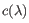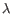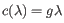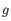## Linear gap cost

If each base inserted or deleted is taxed independently, we have what is known as a linear gap cost model, because the total cost of a gapis proportional to the length of the gap:where the cost per baseis set using the -g/--gapcost parameter. By default, it is set to 3, but you may want to lower that, if you are mapping data from sequencing technology that is prone to produce gaps, such as Ion Torrent, PacBio, or Oxford Nanopore.

If you would like to set the insertion and deletion costs differently, you can do so using a combination of the -g/--gapcost and the -e/--deletioncost parameters.

The -g/--gapcost parameter sets both the insertion and the deletion cost, while the -e/--deletioncost sets deletion cost only. For example, if you would like to set insertion cost to 1 and the deletion cost to 3, you would do the following:

clc_mapper ... -g 1 -e 3 ...

i.e. first set both the deletion and insertion cost to 1 and the set deletion cost back to 3.